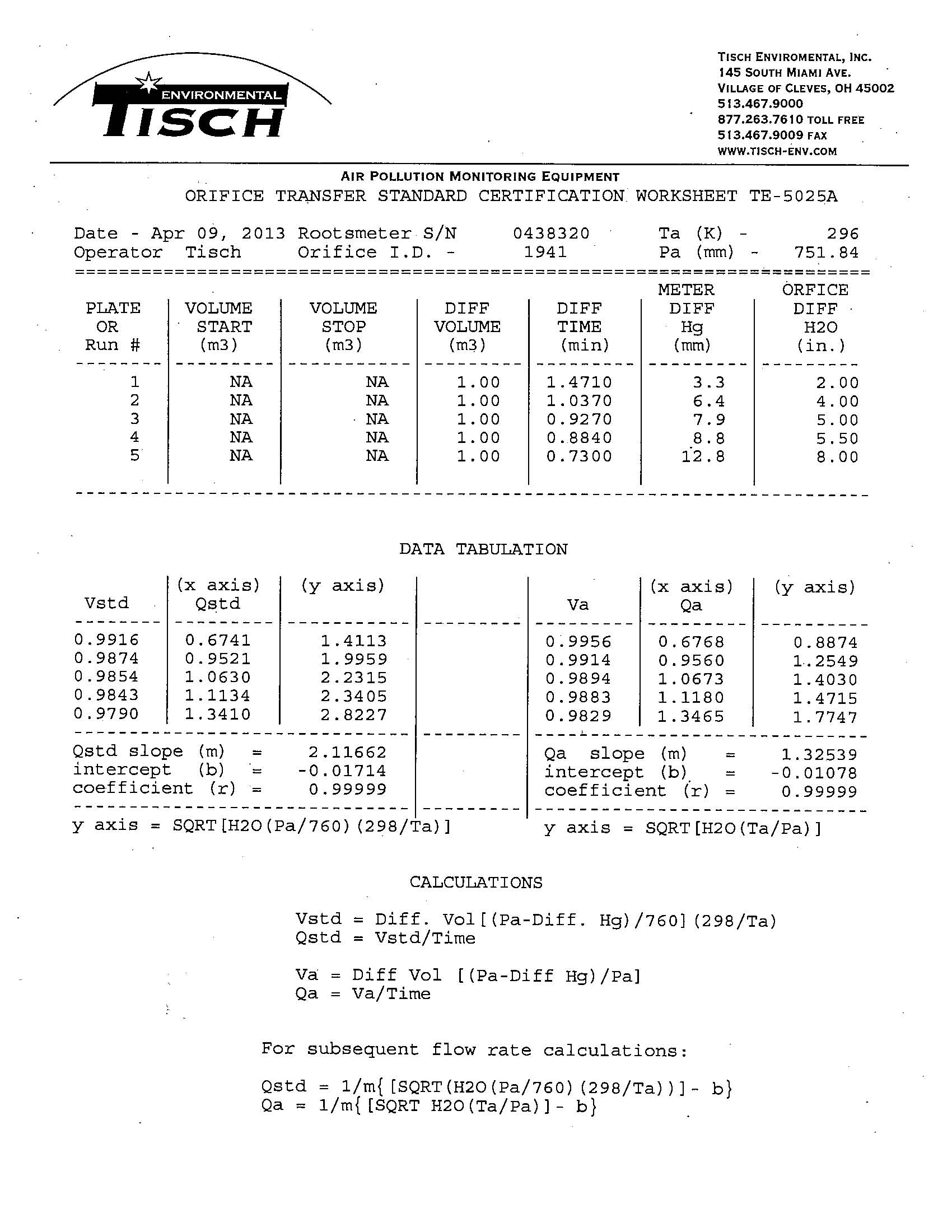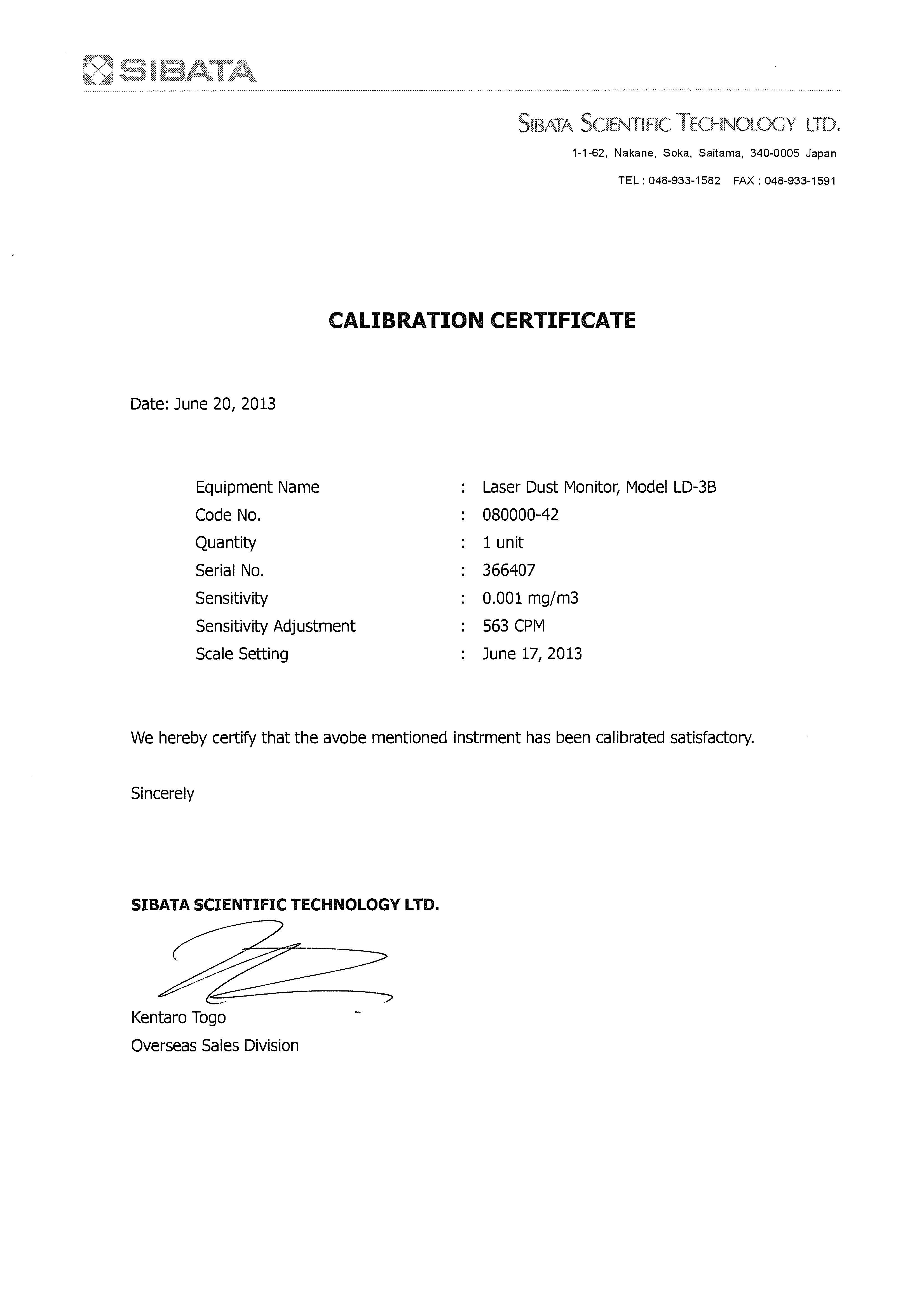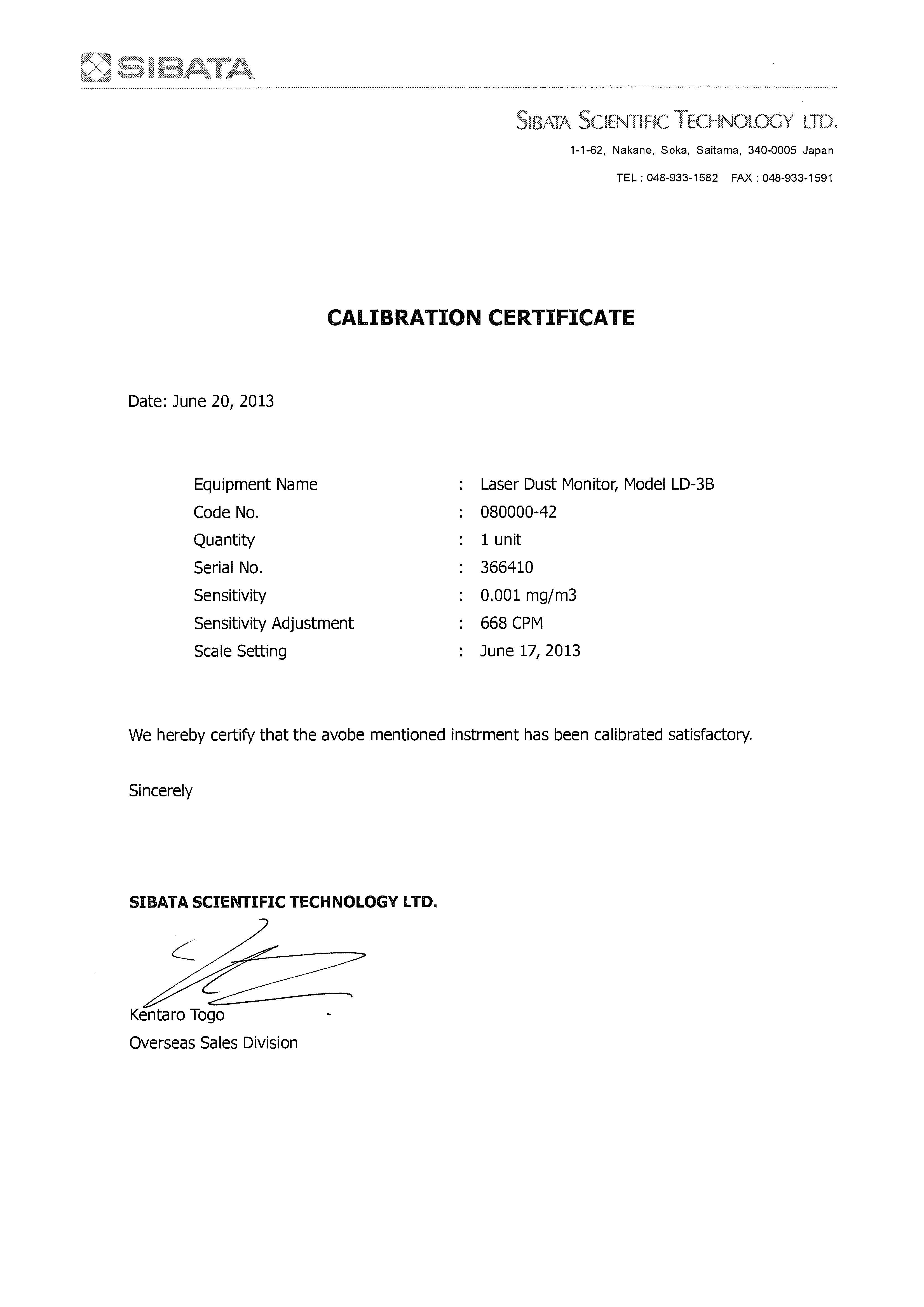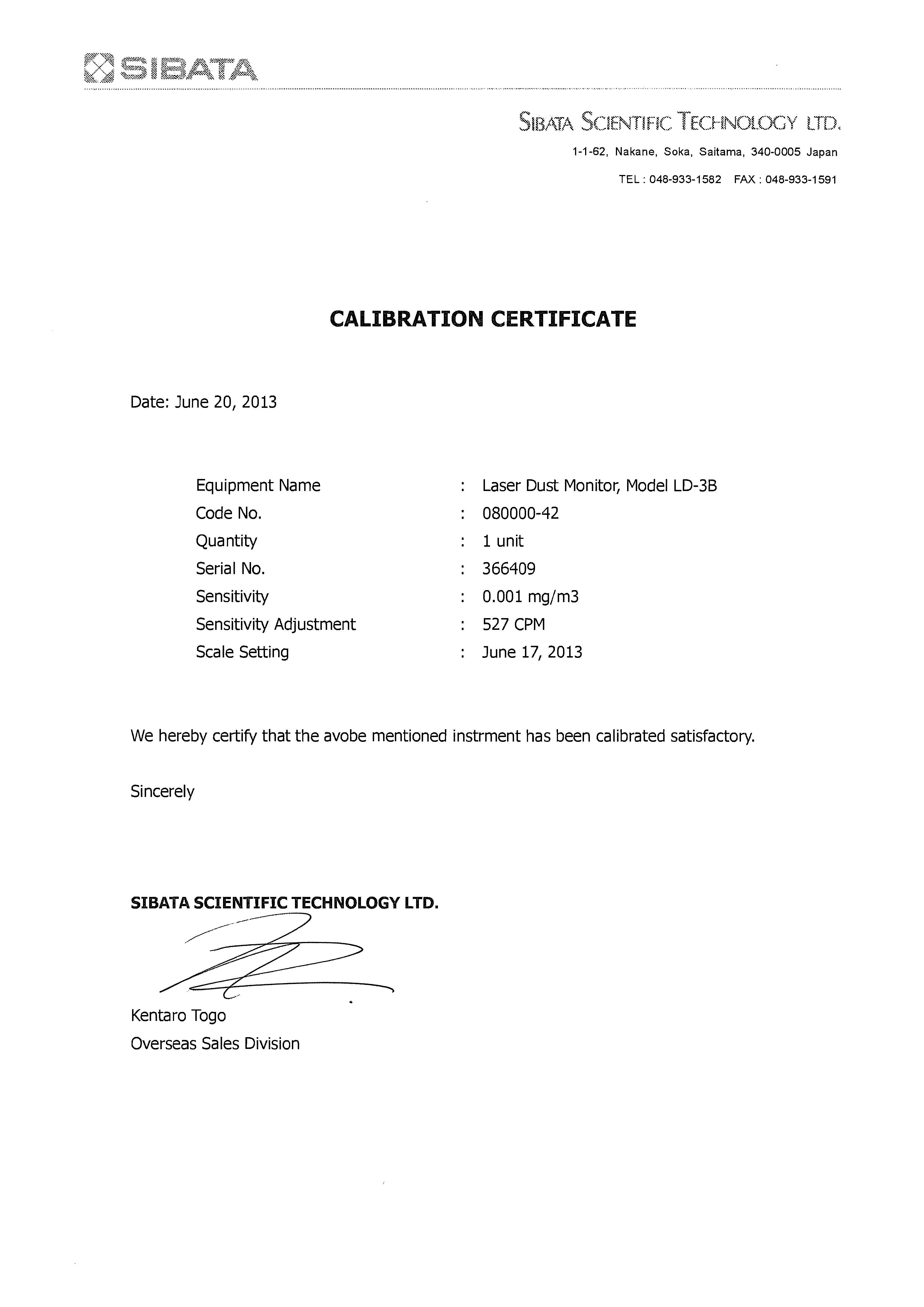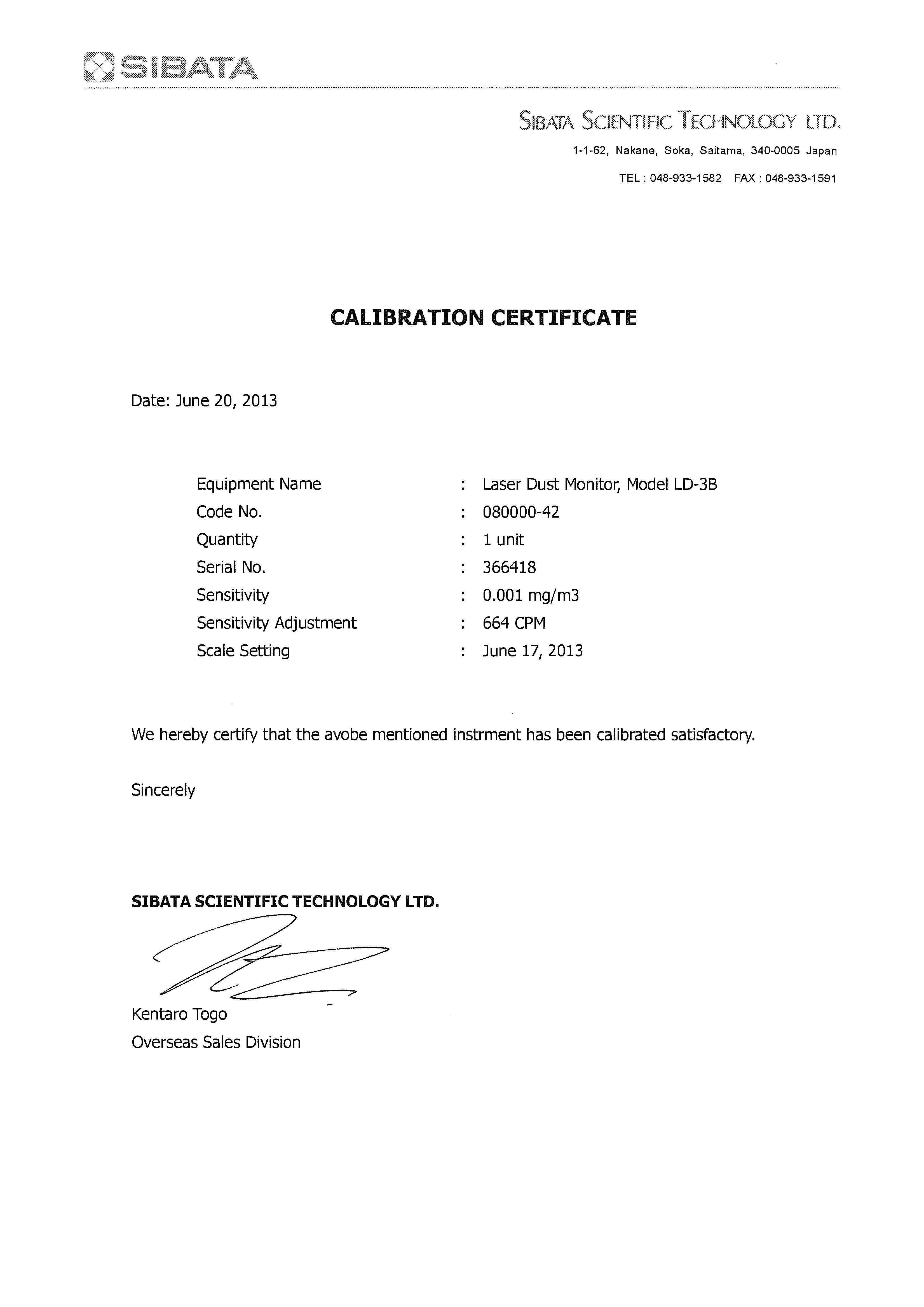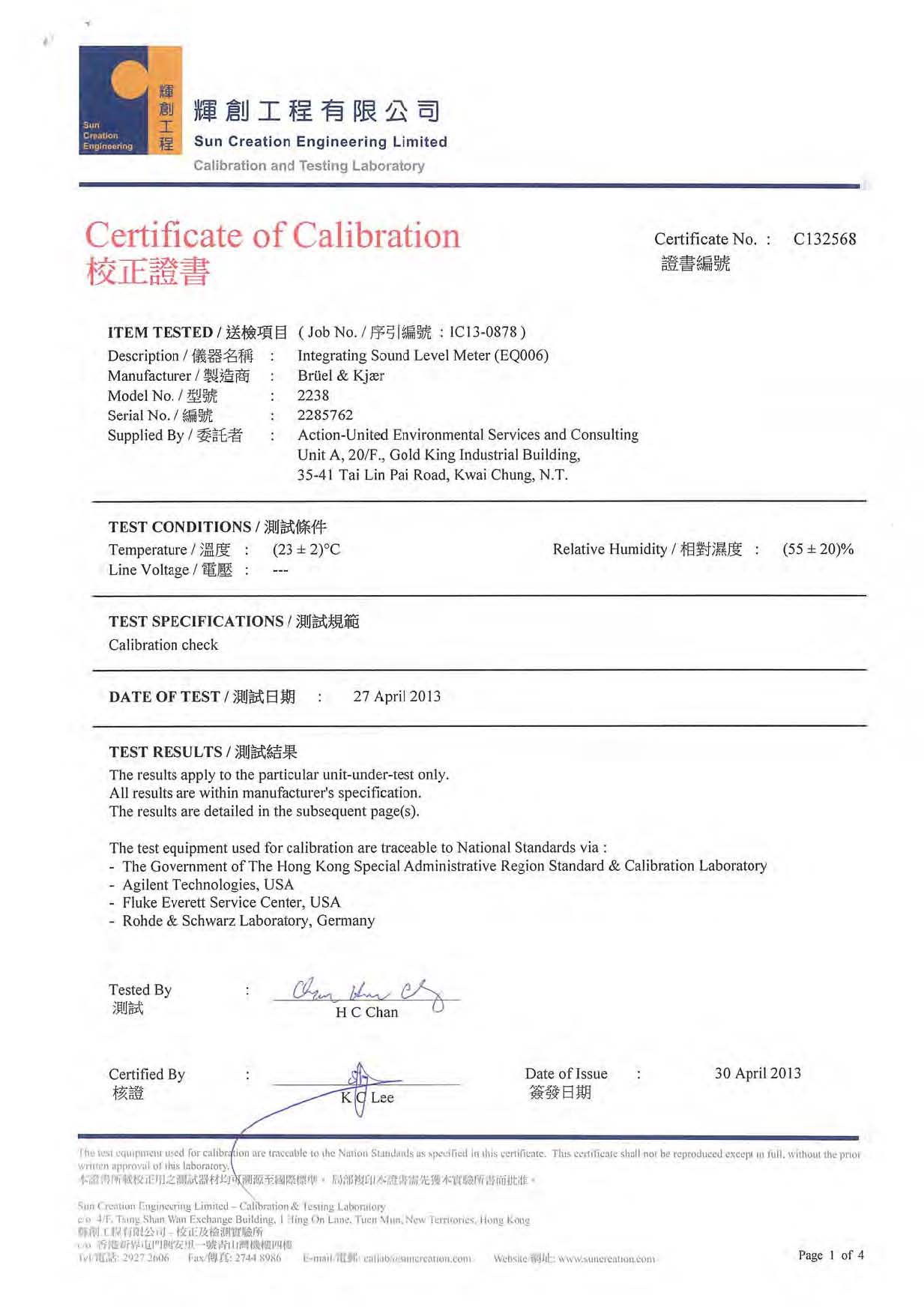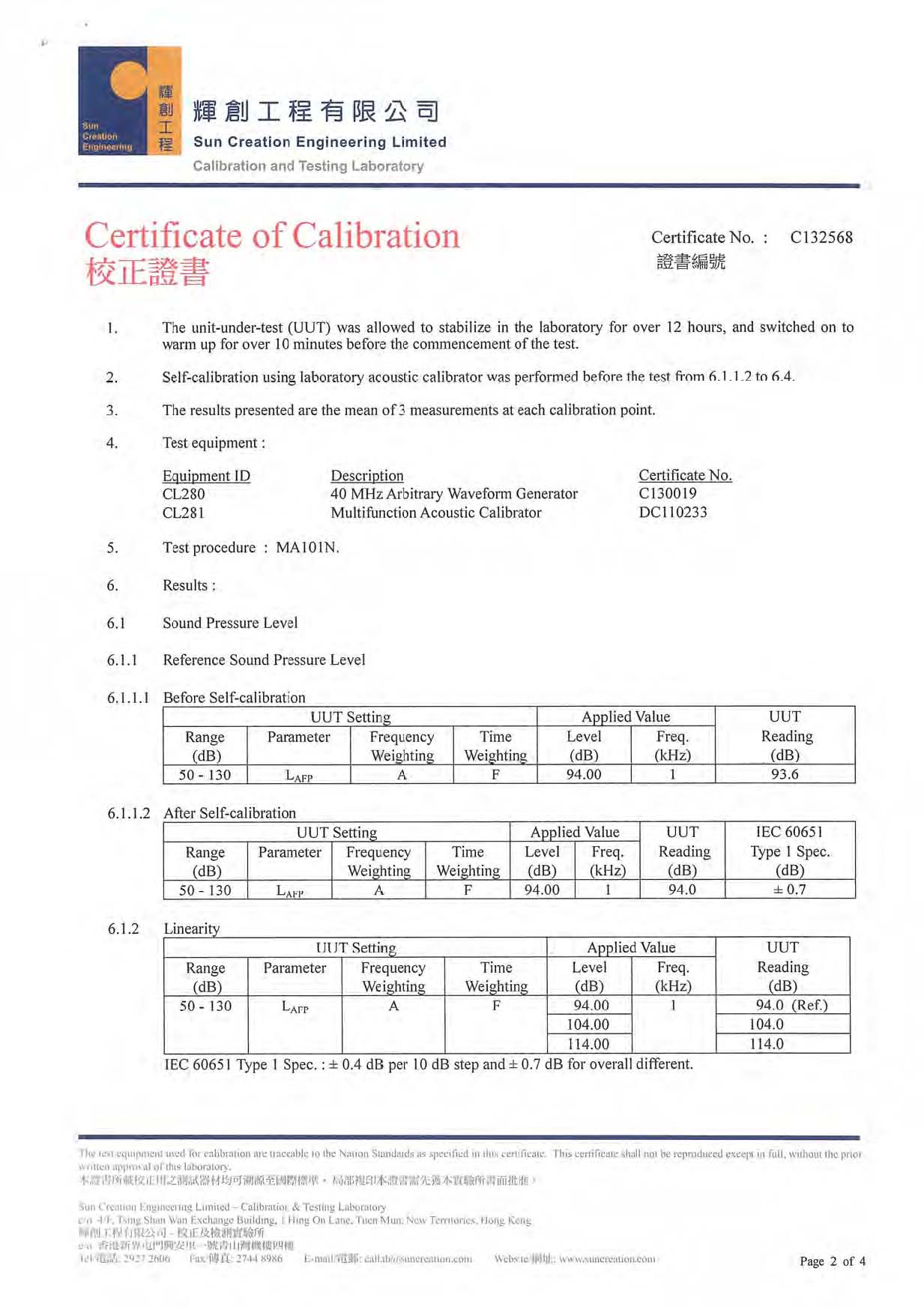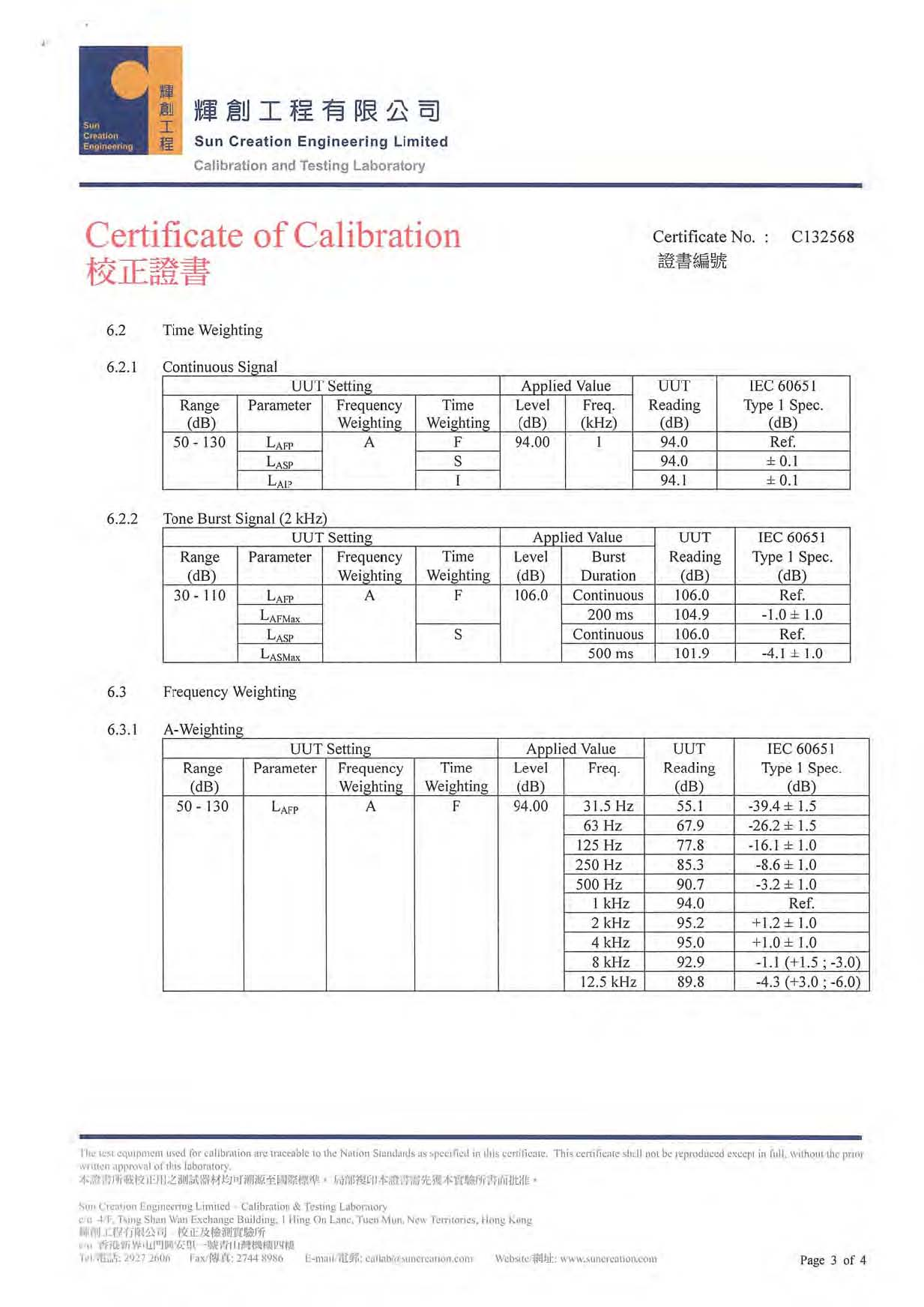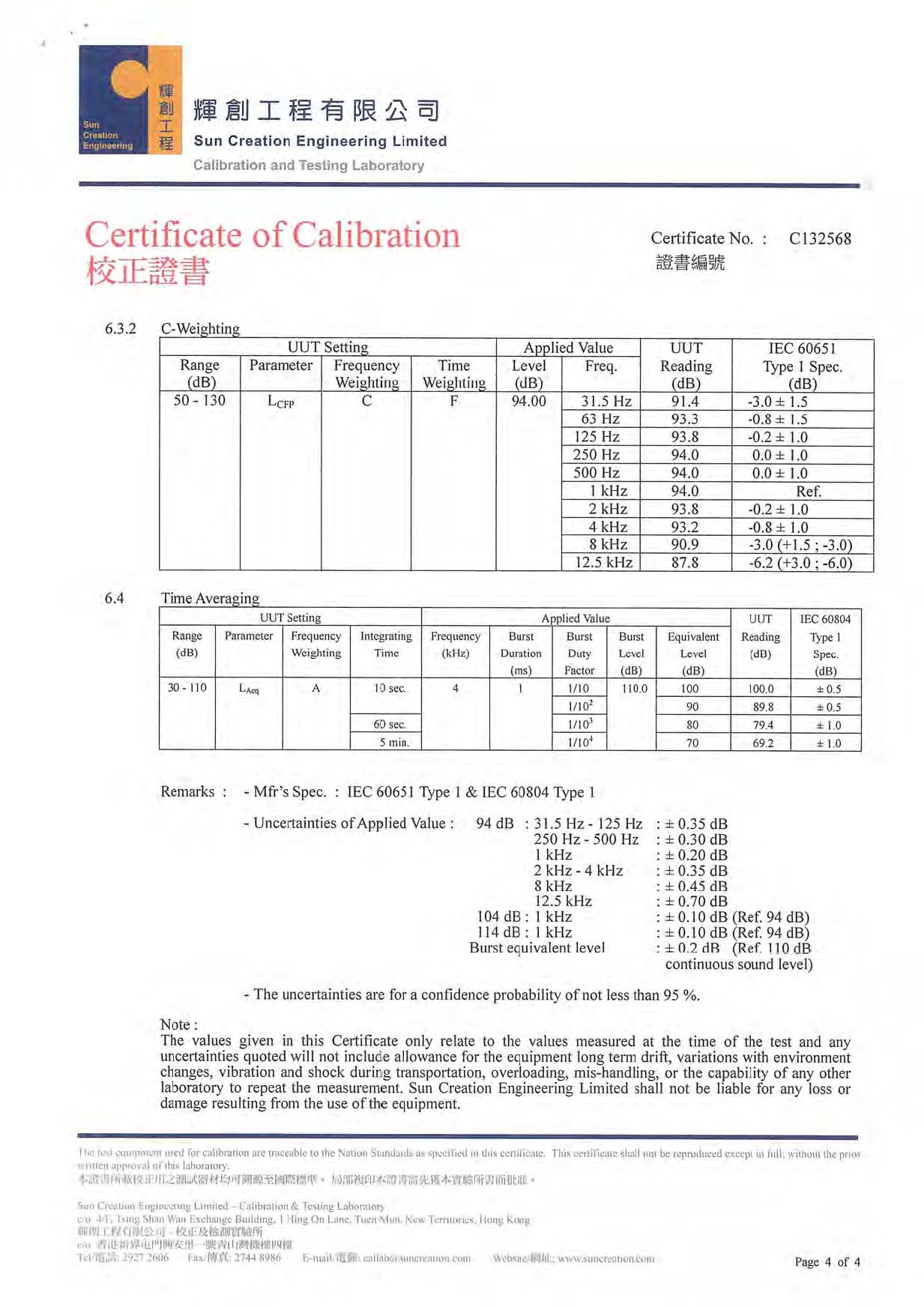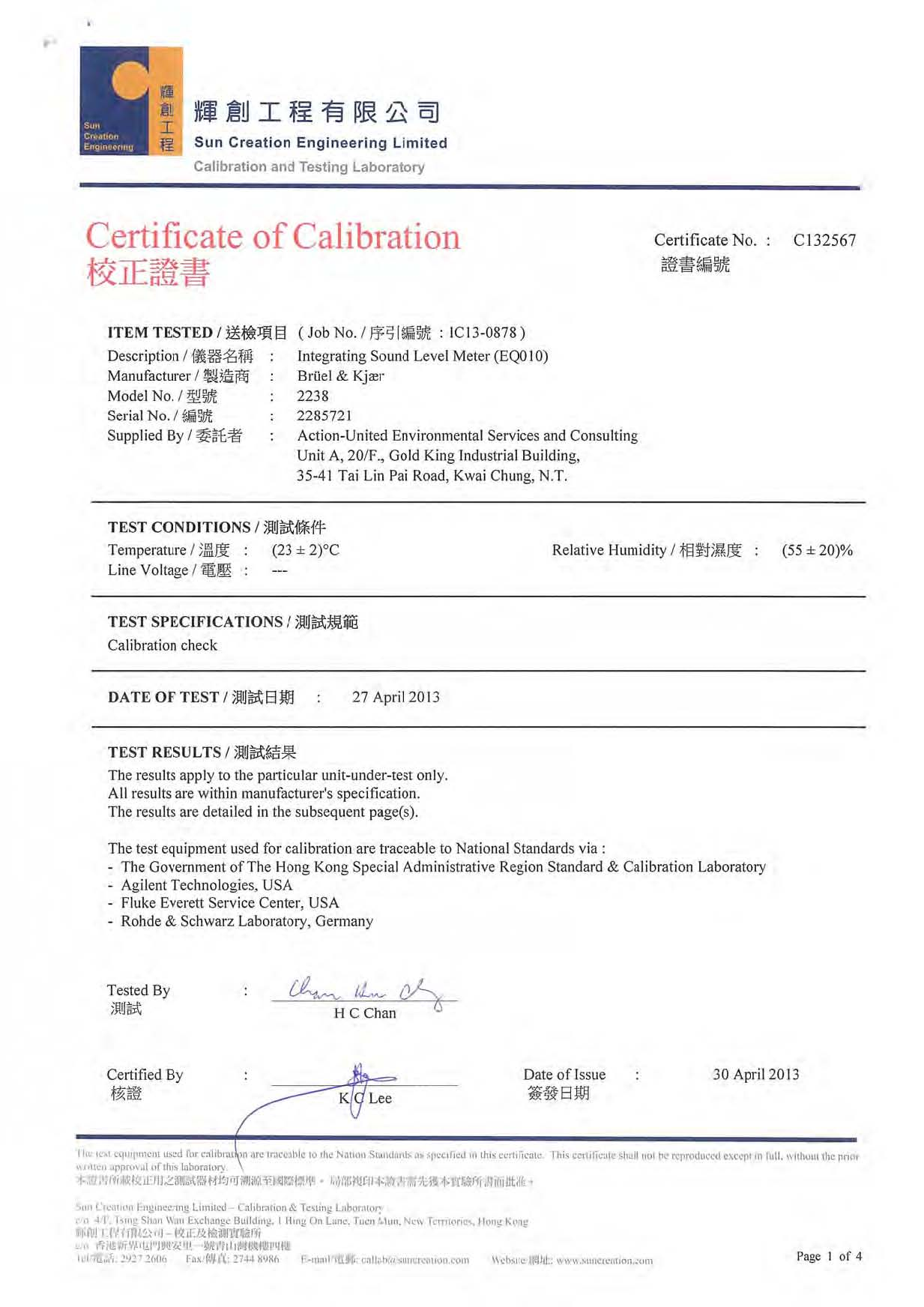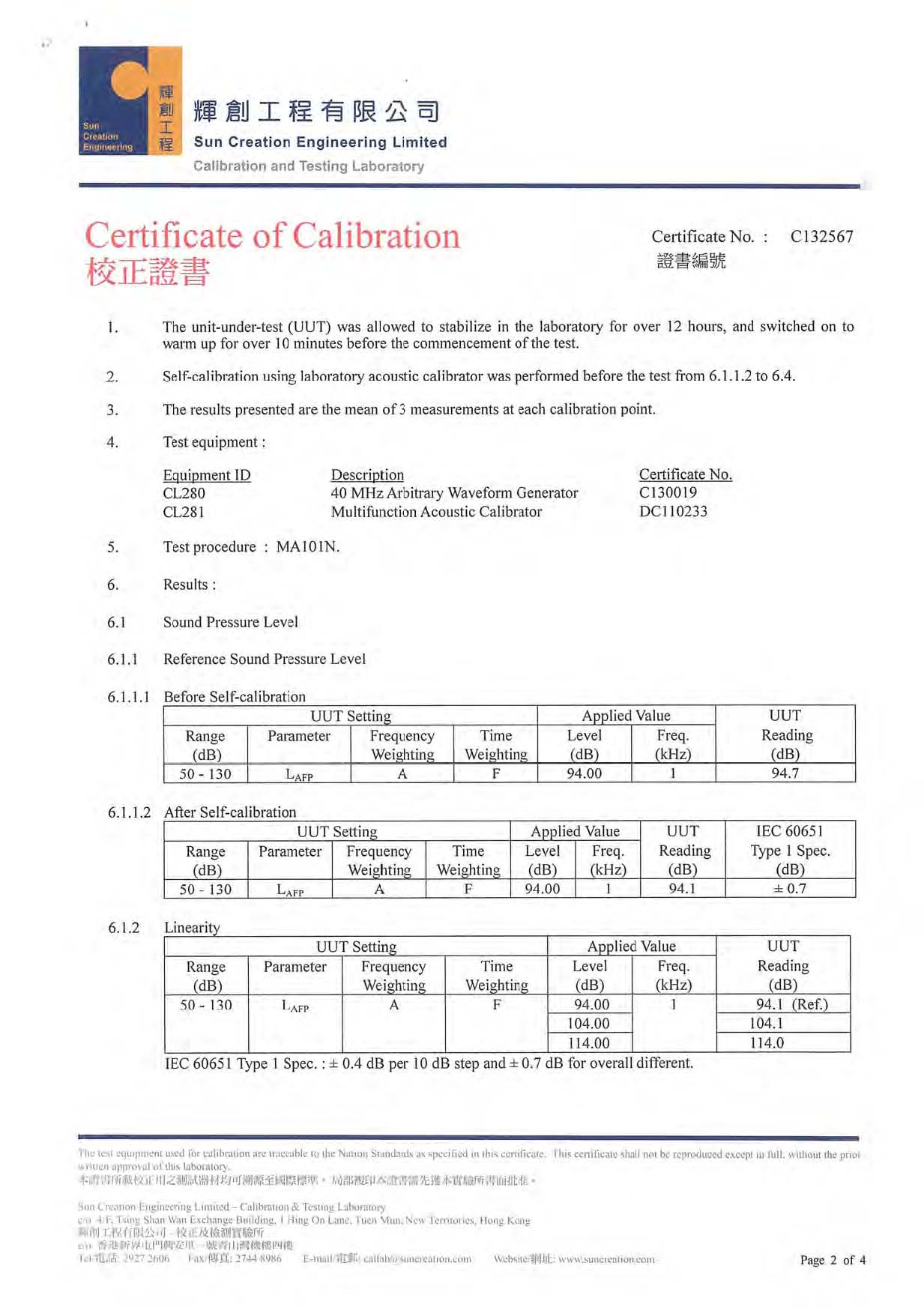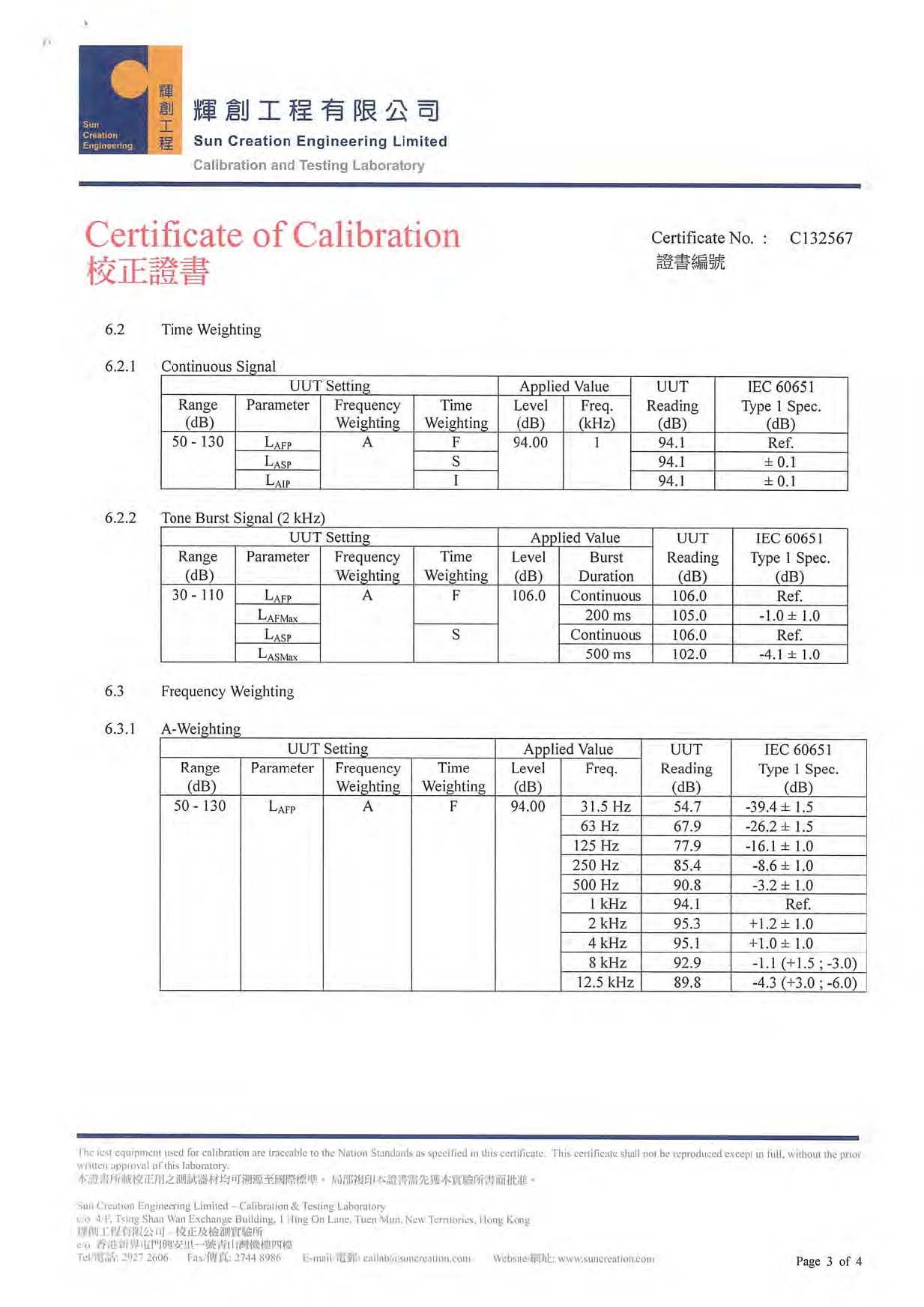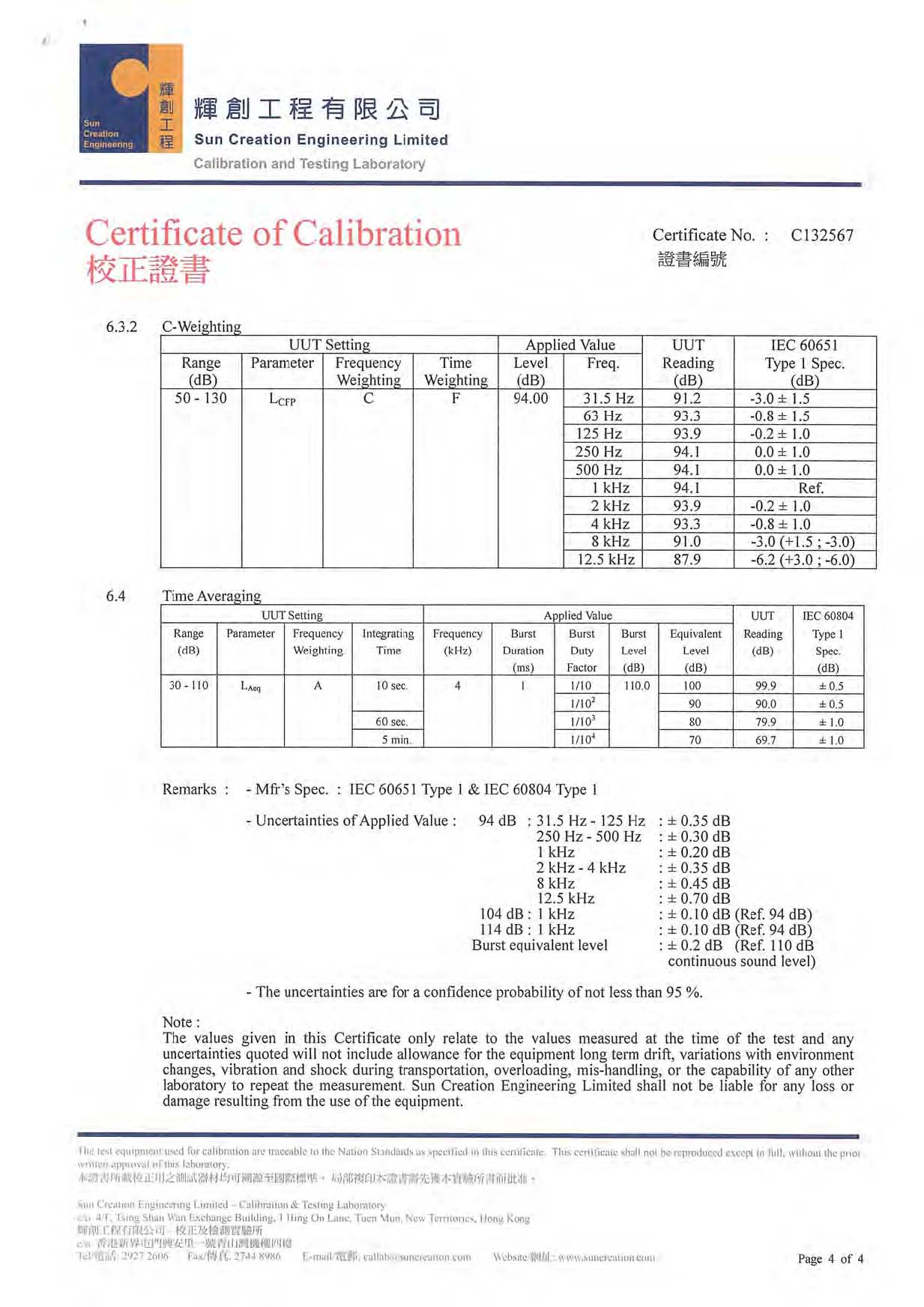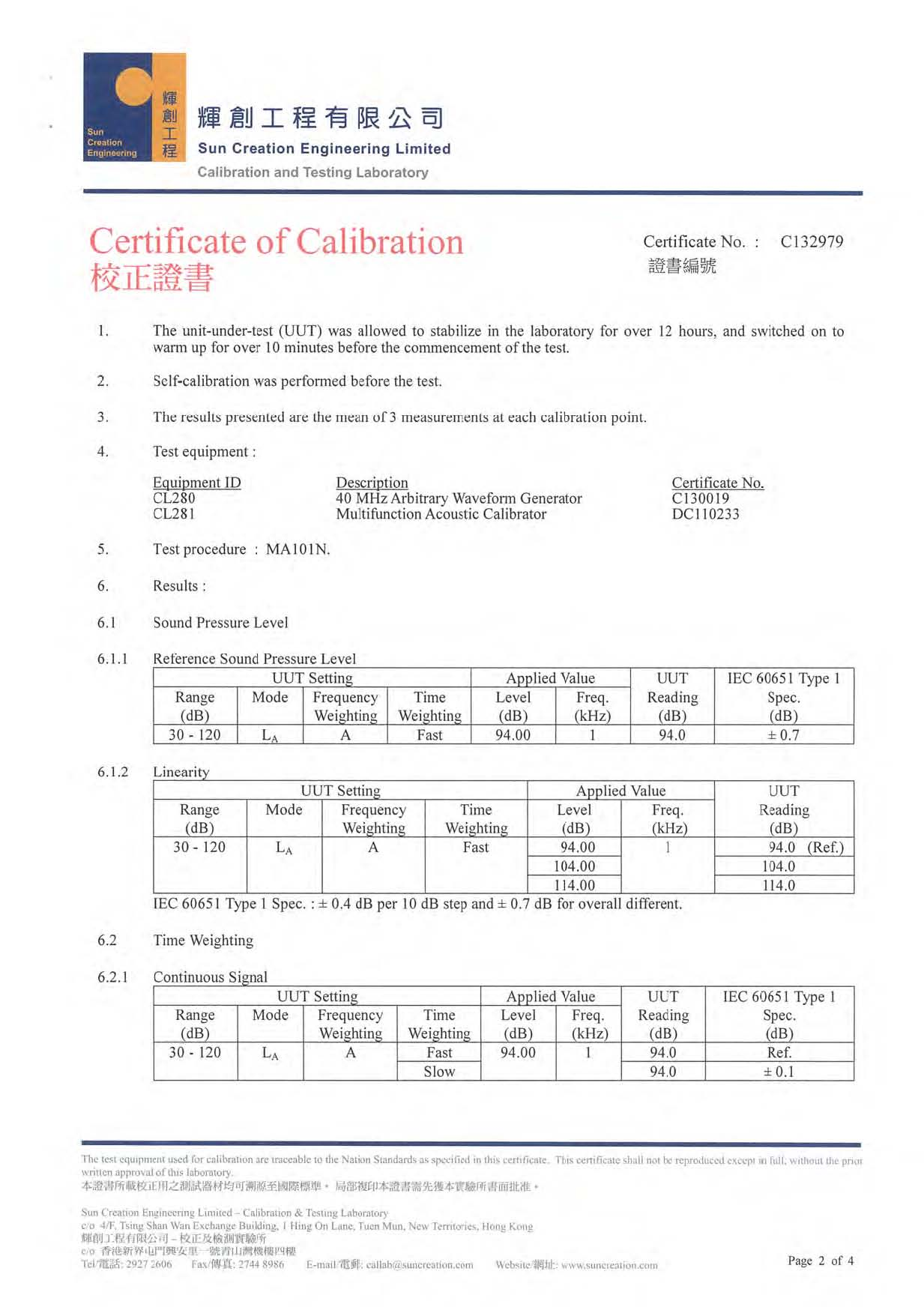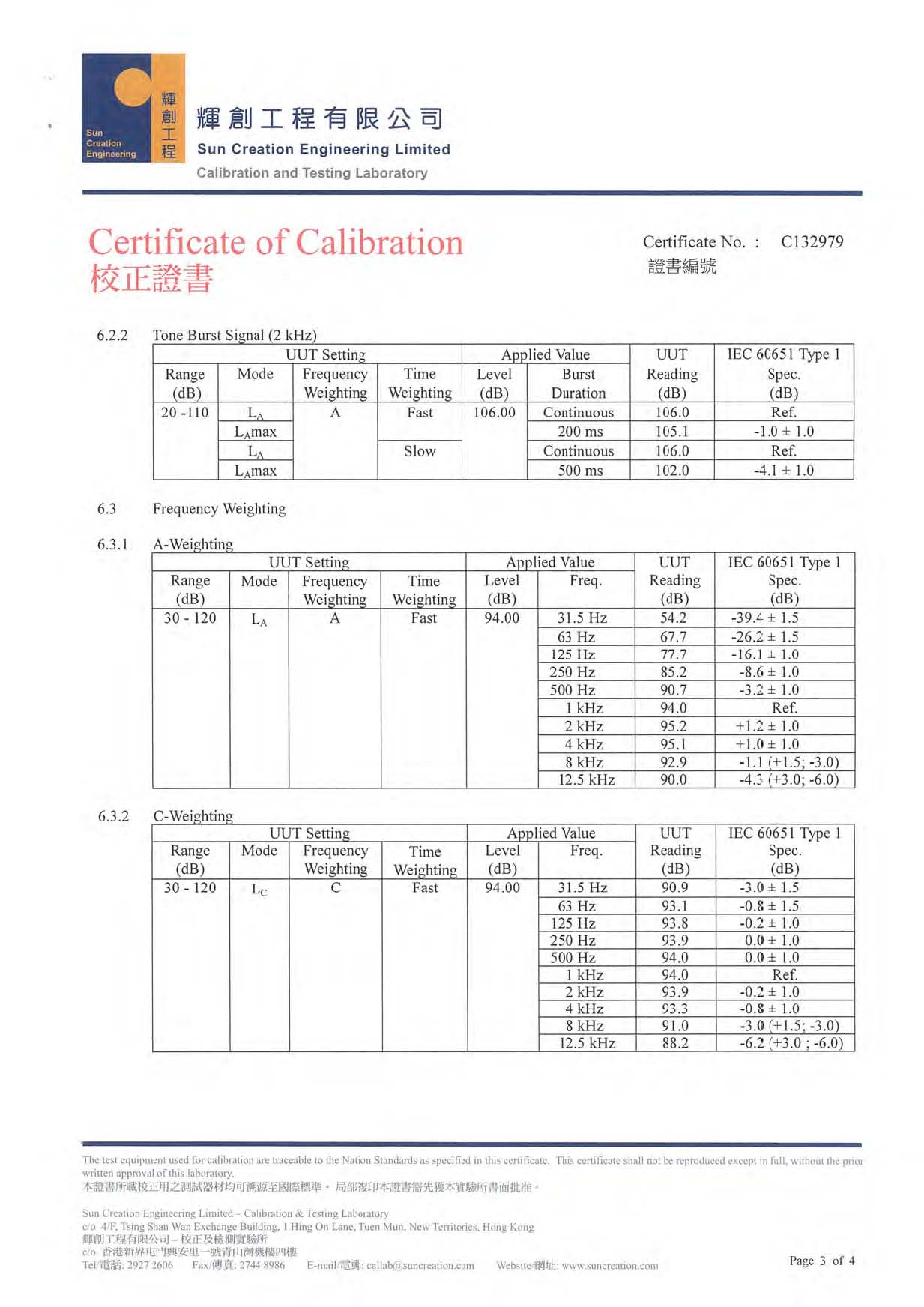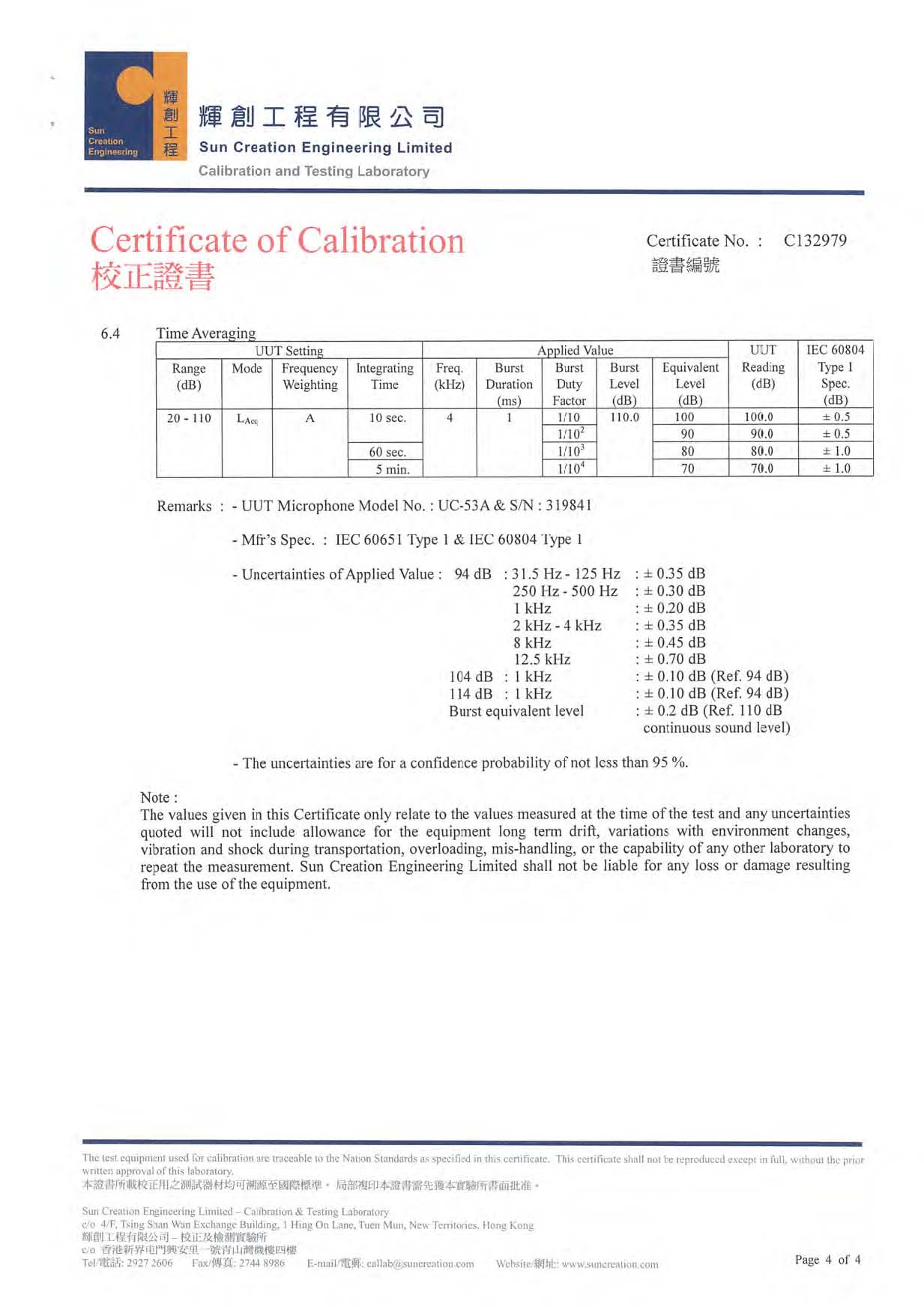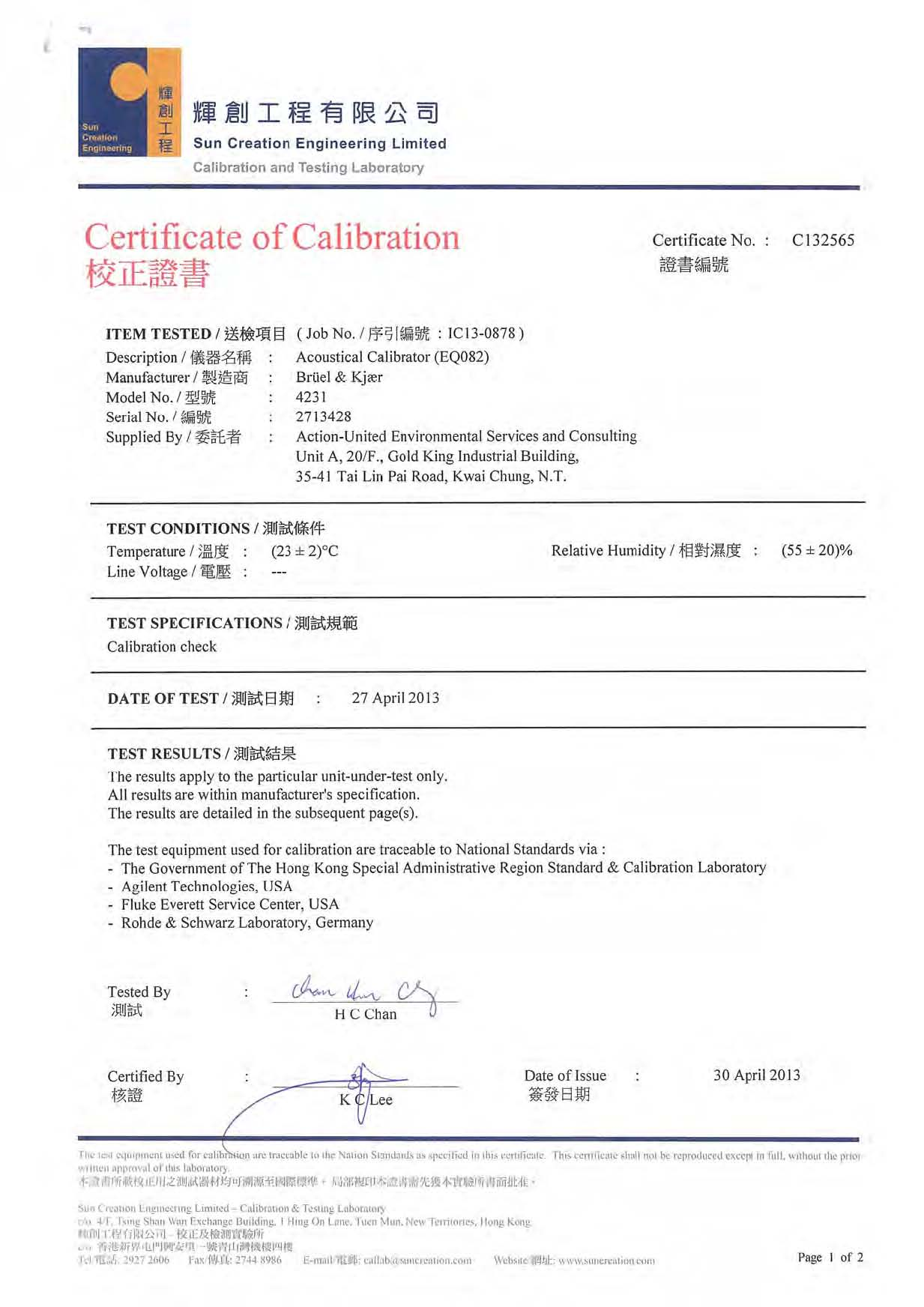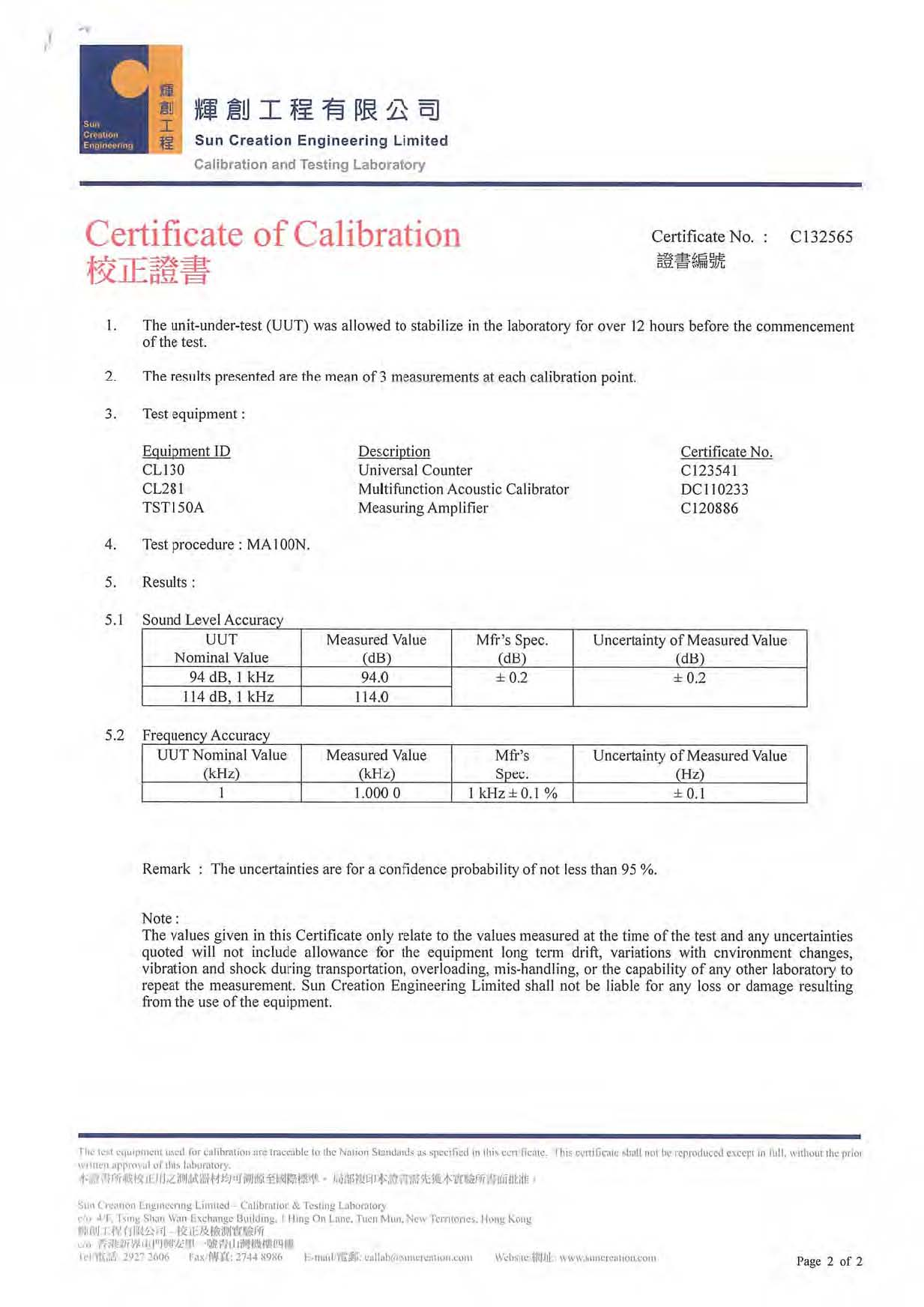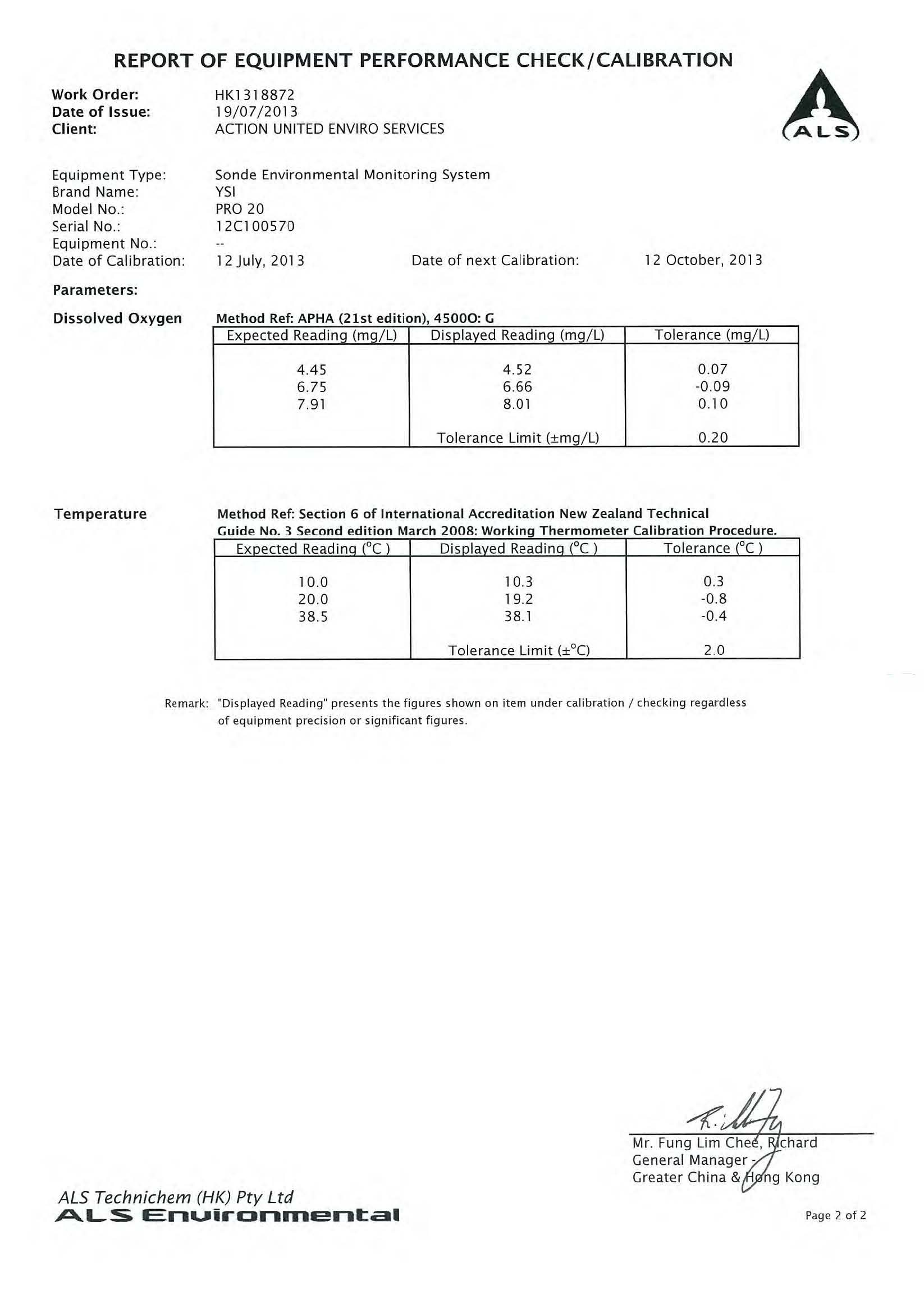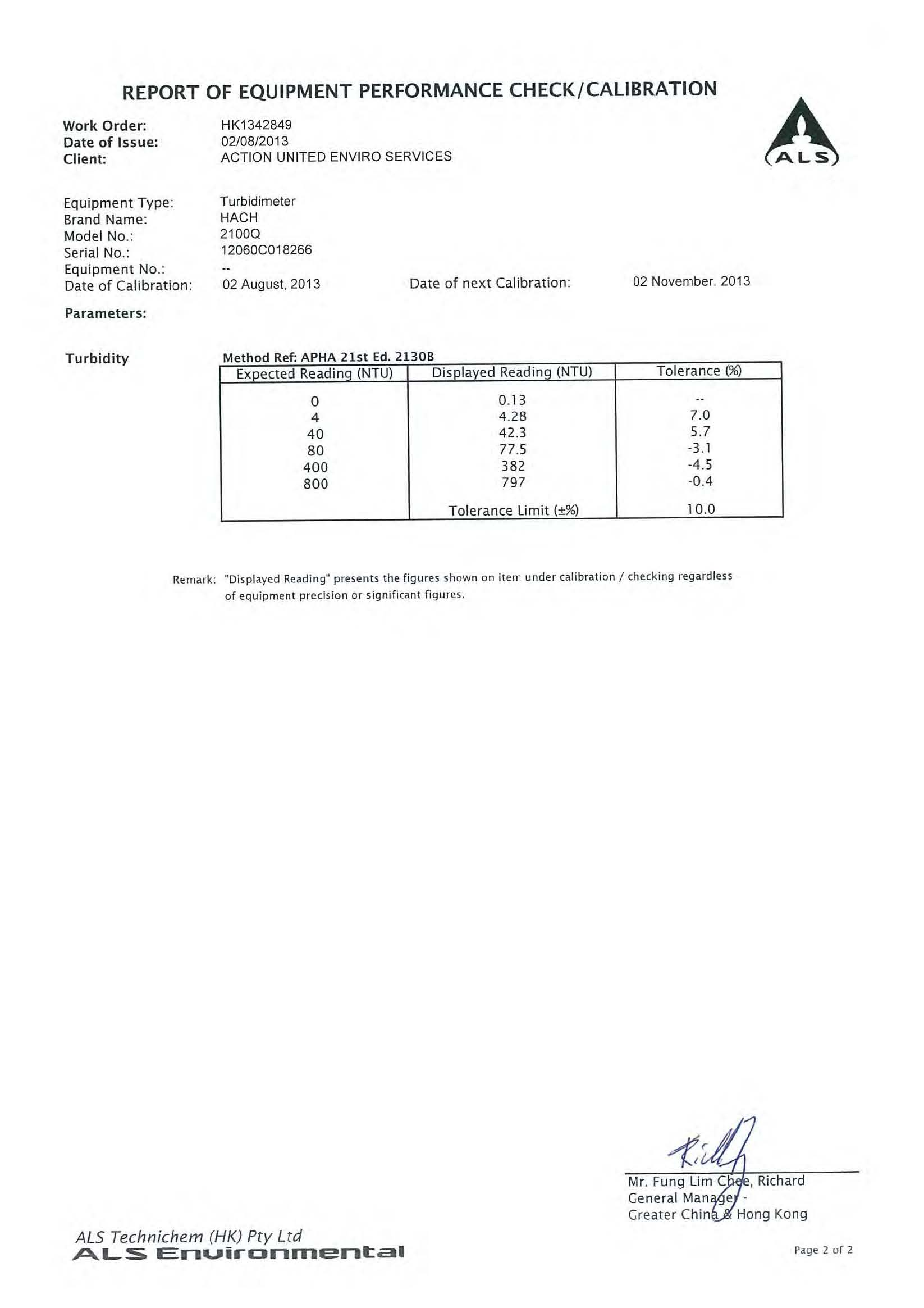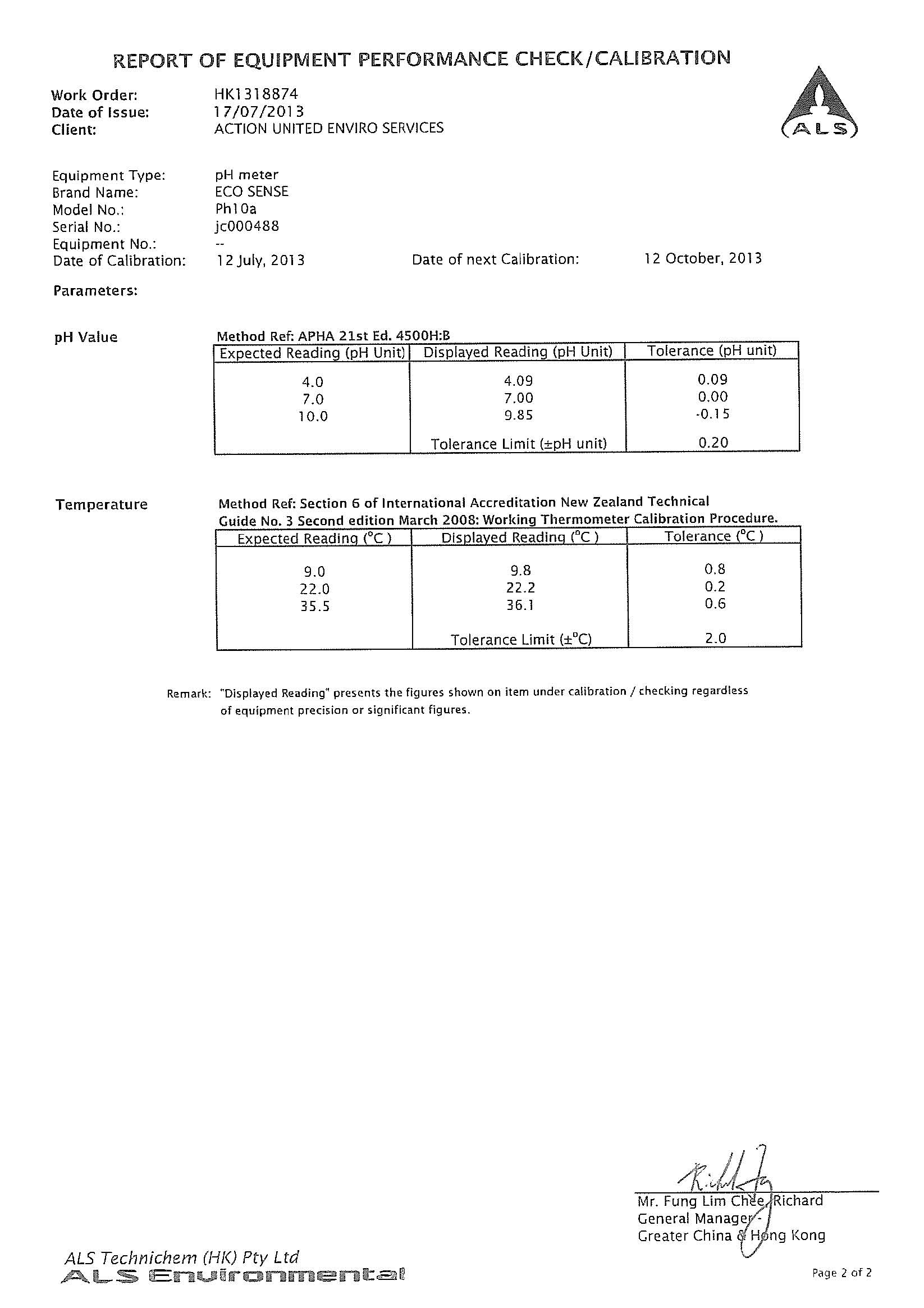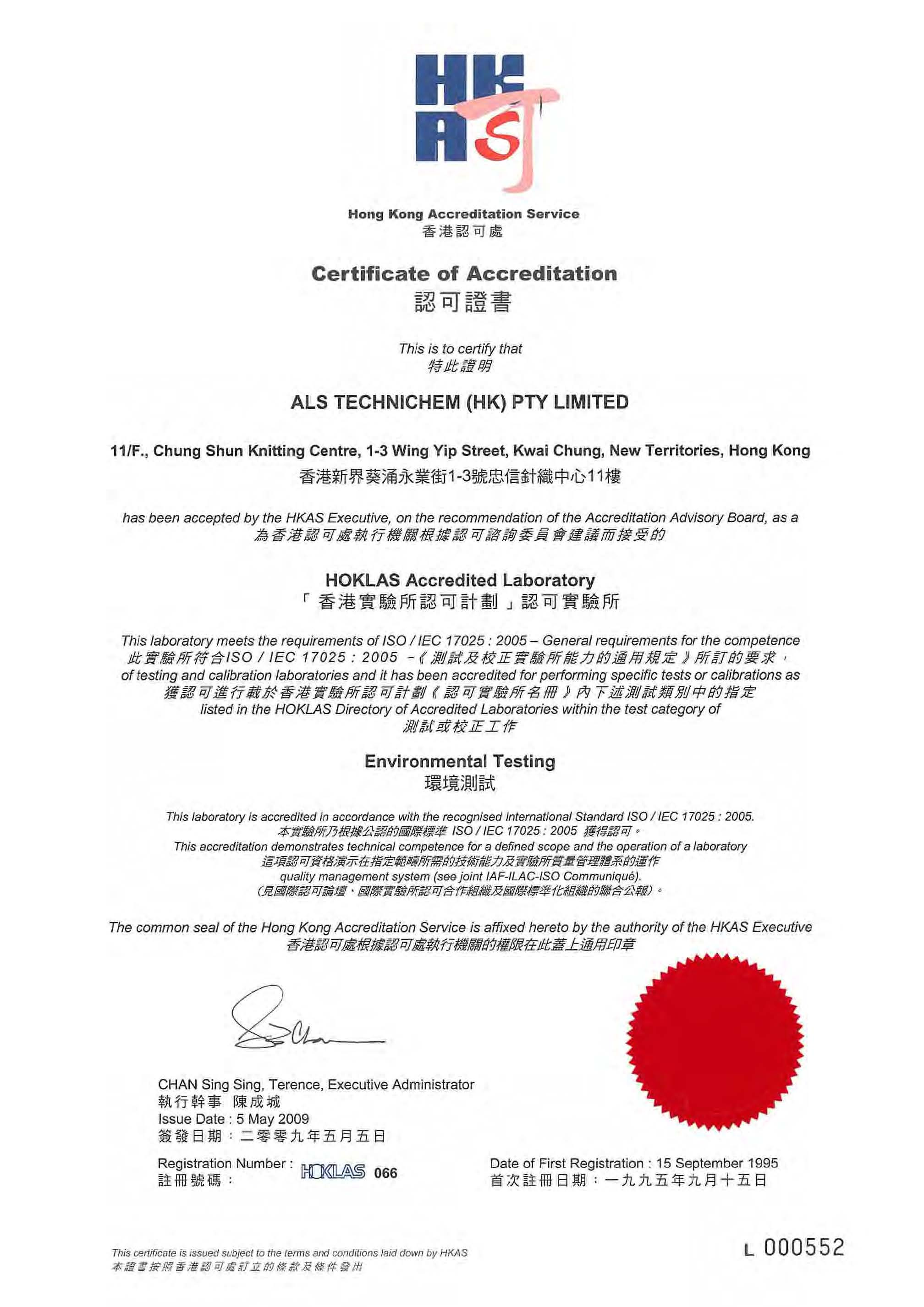Location : Tsung Yuen Ha Village House No. 63 Location ID : AM1 Date of Calibration: 26/8/2013 Next Calibration Date: 26/10/2013 Technician: Ben Tam
CONDITIONS Sea Level Pressure (hPa) 1006.8 Temperature (oC) 28.8 Corrected Pressure (mm Hg) 755.1 Temperature (K) 302
CALIBRATION ORIFICE Make-> TISCH Model-> 5025A Serial # -> 1941 Qstd Slope -> 2.11662 Qstd Intercept -> -0.01714
CALIBRATION
Plate No. H20 (L) (in) H2O (R) (in) H20 (in) Qstd (m3/min) I (chart) IC corrected LINEAR REGRESSION
18 6.8 6.8 13.6 1.734 54 53.15 Slope = 44.9590
13 5.7 5.7 11.4 1.588 45 44.29 Intercept = -26.1666
10 4.7 4.7 9.4 1.443 38 37.40 Corr. coeff. = 0.9960
7 3.4 3.4 6.8 1.228 30 29.53
5 2.5 2.5 5.0 1.054 22 21.65
Calculations : Qstd = 1/m[Sqrt(H20(Pa/Pstd)(Tstd/Ta))-b] IC = I[Sqrt(Pa/Pstd)(Tstd/Ta)] Qstd = standard flow rate IC = corrected chart respones I = actual chart response m = calibrator Qstd slope b = calibrator Qstd intercept Ta = actual temperature during calibration ( deg K ) Pstd = actual pressure during calibration ( mm Hg ) For subsequent calculation of sampler flow: 1/m(( I )[Sqrt(298/Tav)(Pav/760)]-b) m = sampler slope b = sampler intercept I = chart response Tav = daily average temperature Pav = daily average pressure 0.00 10.00 20.00 30.00 40.00 50.00 60.00 0.000 Actual chart response (IC) 0.500 1.000 1.500 2.000 Standard Flow Rate (m3/min) FLOW RATE CHART

Location : Village House near Lin Ma Hang Road Date of Calibration: 26/8/2013 Location ID : AM2 Next Calibration Date: 26/10/2013 Technician: Ben Tam
CONDITIONS Sea Level Pressure (hPa) 1006.8 Corrected Pressure (mm Hg) 755.1 Temperature (oC) 28.8 Temperature (K) 302
CALIBRATION ORIFICE Make-> TISCH Qstd Slope -> 2.11662 Model-> 5025A Qstd Intercept -> -0.01714 Serial # -> 1941
CALIBRATION
Plate No. H20 (L)(in) H2O (R) (in) H20 (in) Qstd (m3/min) I (chart) IC corrected LINEAR REGRESSION
18 13 10 7 5 6.5 5.1 3.6 2 1.6 6.5 5.1 3.6 2 1.6 13.0 10.2 7.2 4.0 3.2 1.695 1.503 1.264 0.944 0.845 55 49 43 35 30 54.13 48.23 42.32 34.45 29.53 Slope = 27.5878 Intercept = 7.2413 Corr. coeff. = 0.9966
Calculations : Qstd = 1/m[Sqrt(H20(Pa/Pstd)(Tstd/Ta))-b] IC = I[Sqrt(Pa/Pstd)(Tstd/Ta)] Qstd = standard flow rate IC = corrected chart respones I = actual chart response m = calibrator Qstd slope b = calibrator Qstd intercept For subsequent calculation of sampler flow: 1/m(( I )[Sqrt(298/Tav)(Pav/760)]-b) m = sampler slope b = sampler intercept I = chart response Tav = daily average temperature Pav = daily average pressure 0 10 20 30 40 50 60 0 0.5 1 1.5 2 Actual chart response (IC) Standard Flow Rate (m3/min) FLOW RATE CHART